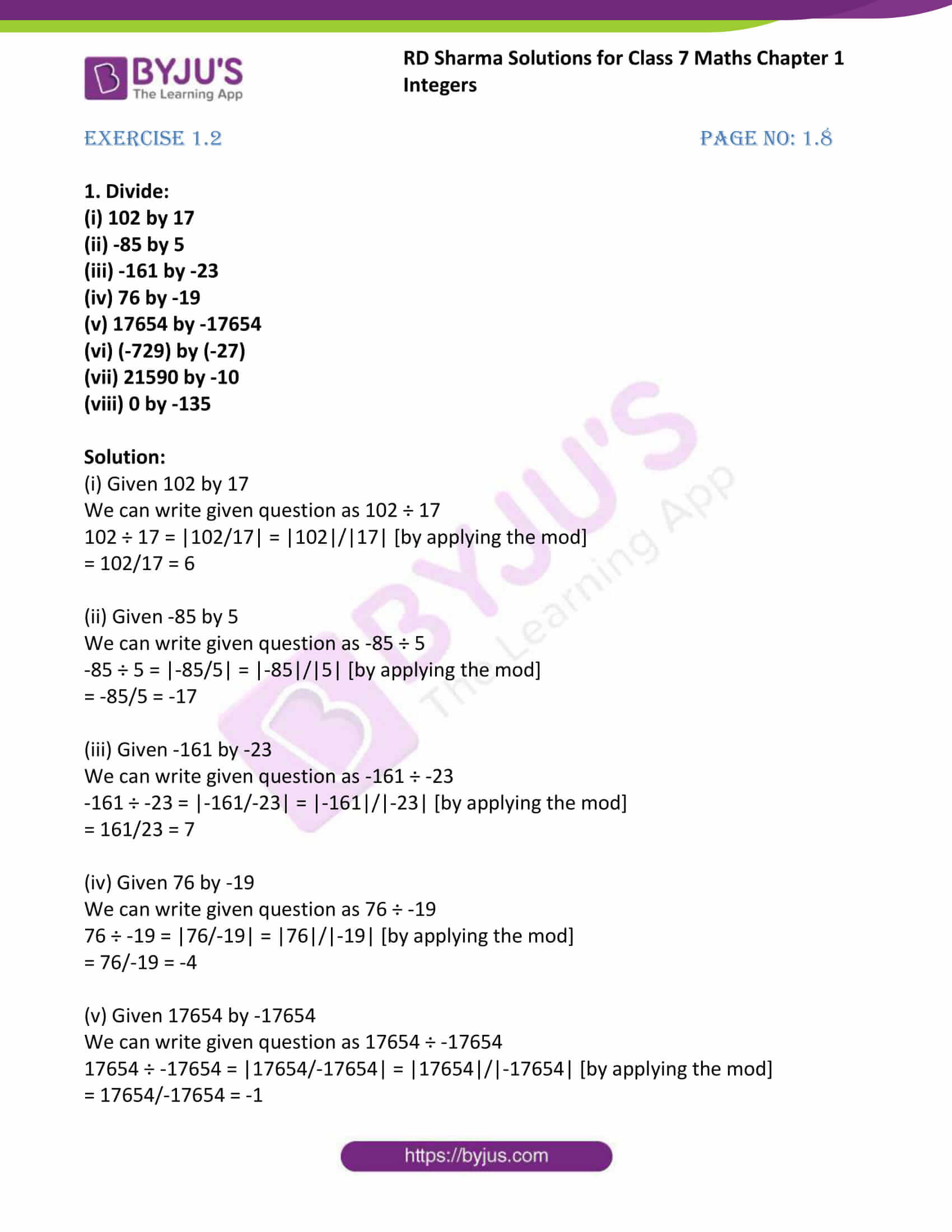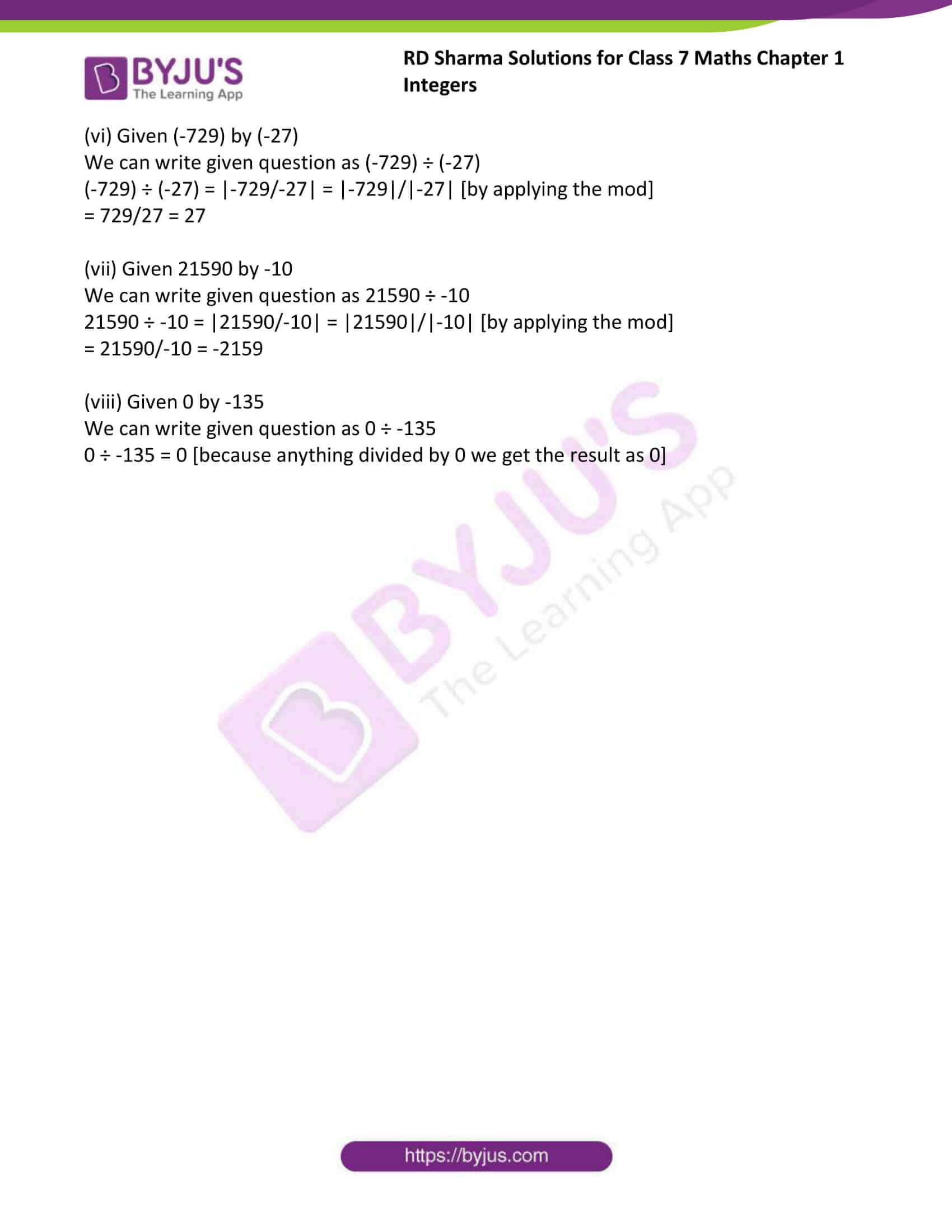# RD Sharma Solutions For Class 7 Maths Chapter 1 Integers Exercise 1.2

Students can refer and download the RD Sharma Solutions for Class 7 Chapter 1 Integers Exercise 1.2 from the link provided here. Our experts have solved the RD Sharma Solutions to ensure that the students are thorough with their concepts by practising the solutions. The solutions PDF can be used as a model of reference by the students to improve their conceptual knowledge and understand the different ways used to solve the problems. In this exercise, we shall study the concept of division of integers and their properties. By practising RD Sharma Solutions for Class 7, students will be able to grasp the concepts perfectly. It also helps in boosting their confidence, which plays a crucial role in the examinations.

## Download the PDF of RD Sharma Solutions For Class 7 Maths Chapter 1 – Integers Exercise 1.2### Access answers to Maths RD Sharma Solutions For Class 7 Chapter 1 – Integers Exercise 1.2

Exercise 1.2 Page No: 1.8

1. Divide:

(i) 102 by 17

(ii) -85 by 5

(iii) -161 by -23

(iv) 76 by -19

(v) 17654 by -17654

(vi) (-729) by (-27)

(vii) 21590 by -10

(viii) 0 by -135

Solution:

(i) Given 102 by 17

We can write given question as 102 ÷ 17

102 ÷ 17 = |102/17| = |102|/|17| [by applying the mod]

= 102/17 = 6

(ii) Given -85 by 5

We can write given question as -85 ÷ 5

-85 ÷ 5 = |-85/5| = |-85|/|5| [by applying the mod]

= -85/5 = -17

(iii) Given -161 by -23

We can write given question as -161 ÷ -23

-161 ÷ -23 = |-161/-23| = |-161|/|-23| [by applying the mod]

= 161/23 = 7

(iv) Given 76 by -19

We can write given question as 76 ÷ -19

76 ÷ -19 = |76/-19| = |76|/|-19| [by applying the mod]

= 76/-19 = -4

(v) Given 17654 by -17654

We can write given question as 17654 ÷ -17654

17654 ÷ -17654 = |17654/-17654| = |17654|/|-17654| [by applying the mod]

= 17654/-17654 = -1

(vi) Given (-729) by (-27)

We can write given question as (-729) ÷ (-27)

(-729) ÷ (-27) = |-729/-27| = |-729|/|-27| [by applying the mod]

= 729/27 = 27

(vii) Given 21590 by -10

We can write given question as 21590 ÷ -10

21590 ÷ -10 = |21590/-10| = |21590|/|-10| [by applying the mod]

= 21590/-10 = -2159

(viii) Given 0 by -135

We can write given question as 0 ÷ -135

0 ÷ -135 = 0 [because anything divided by 0 we get the result as 0]

## RD Sharma Solutions for Class 7 Maths Chapter – 1 Integers Exercise 1.2

Exercise 1.2 of RD Sharma Solutions for Chapter 1, Integers deals with the basic concepts related to Integers and fundamental operations on it. Some of the topics focused prior to Exercise 1.2 are listed below.

• Division of Integers
• Properties of division